Tableau allows us to harness the power of our data. So, it seems fitting to explore one of the many ways to do it. After all, it is no use having access to a multitude of data without being able to understand and apply it!

In this tutorial, we will explore moving calculations and wrap around. First, let’s depict a scenario and second let’s learn how to do it using step-by-step instructions using Tableau. Of course, it is important to note that this blog is intended for those who are already somewhat familiar with the inner workings of Tableau.

First, let’s refresh.

What are Moving Calculations?

Tableau defines this as “determines the value for a mark in the view by performing an aggregation (sum, average, minimum, or maximum) across a specified number of values before and/or after the current value.”

What exactly does that mean? Let’s translate it. A moving calculation allows insight into a subsection of the entire data, within a specified period. In business, that can be sales totals over a while, or perhaps insights into stock trends.

# Applied to a Scenario:

Applying moving calculations and wrap around to a set of data, it is possible to infer possible causes of road incidents. To start, we can plot the number of incidents per hour with a moving average of 3 hours charted above it.

In Figure 1, time is plotted on the X-axis,  whereas the Y-axis represents the number of incidents. Now, imagine that we would like to create a moving window. We will start with a specified time and the following two hours. The line above in the chart is the quick table calculation – the moving SUM (plotted on the secondary Y-axis).

Figure 1:In Figure 2, this method displays the default SUM generated by Tableau. We can identify that the maximum number of incidents falls within the window of 18:00 to 21:00.

Figure 2 & 3: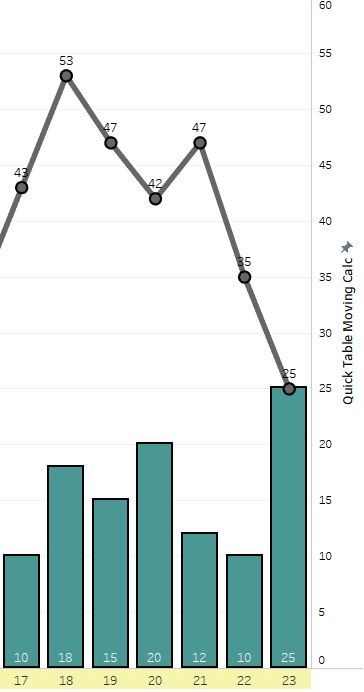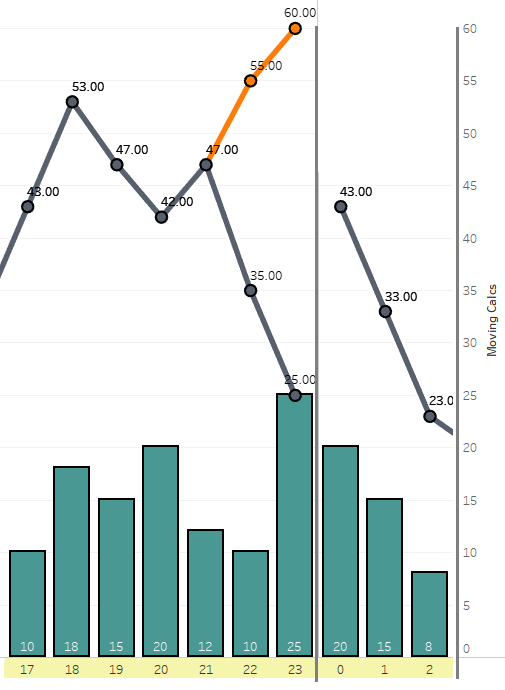Figure 2 lacks wrap around. With this in mind, it looks as though the 3 hours containing the most traffic incidents matches up nicely with the evening rush hour period. The potential causes at fault being fatigue and traffic congestion.

The built-in Moving calculations from Tableau’s Quick Table calculation considers fewer data points at either end, than it does in the middle of the range.

In this scenario, it is necessary to observe data beyond the confines of a single day.  We have to create wrap around to observe the full picture. This should include first values (as Next) or last values (as Previous) in the moving calculations.

In Figure 3, wrap around has been added. Now that there is data displayed as a rolling time period, the results have changed. We see that the actual time period with the most incidents is from 23:00 until 2:00 (11pm – 2am). Knowing this, the reason could change from traffic congestion to factors such as alcohol consumption, speeding, reduced visibility and fatigue.

# Other Applications

Think of the span of a year and seasonal patterns. Of course, seasons do not respect the confines of human manufactured time, so we need to go beyond a Quick Table Calculation. If we were to track temperatures or snow accumulation during winter the results would start in one year and complete in the next.

So, how can wrap around be done?

# How to Compute

Going back to Figure 2, the last two moving sums only consider up to the point of 23:59. This eliminates the possibility of “Current hour plus the following two” and preventing  wrap around at midnight.

We are going to build the calculation to include the wrapped around counts to get accurate results.

What we will need are: The current position (INDEX()), the size determined by the scope (SIZE()) and the moving window size here I have hardcoded 2 when above screen shots were taken. But a parameter [Moving Window Span] can be used to make the moving window size dynamic.

Let’s define Index and Size to utilize in our Moving Calculation.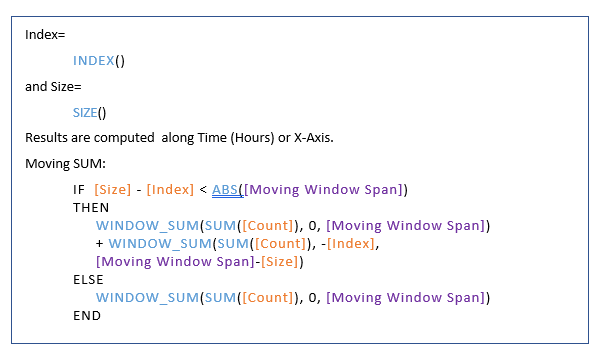In simple terms, at each line (Index)

• If there are sufficient data points for moving calculation, i.e. current position is less than window span away from last row (Size – Index >= Span parameter) Then the usual moving calculation – the Quick Table Calculation – works well Count from Current (0) to next (Moving Window Span) will return expected values.
• If the data points remaining (Size – Index) are fewer than the window span for moving sum, pick up whatever points are there till the last one – Clause 1 of the Else condition above And then add remaining ones from the beginning of the Time axis. Starting from FIRST(): Index being the current position, Minus Index will be first data point. Until <Span> number of values are covered: (Moving Window Span Minus Size)

And of course, the important point to remember – When working with Table Calculations – is the SCOPE and DIRECTION of Table Calculation.

All these (Index, Size and Window_SUM) are to be calculated Along Time (Hours).

Note: The calculation will not return correct results if the scope is incorrect.

If you would like to get in-depth – allowing the parameter to be negative (to use Previous n values instead of Next n); the final calculation (Wrap Around Moving Calc) will look like this: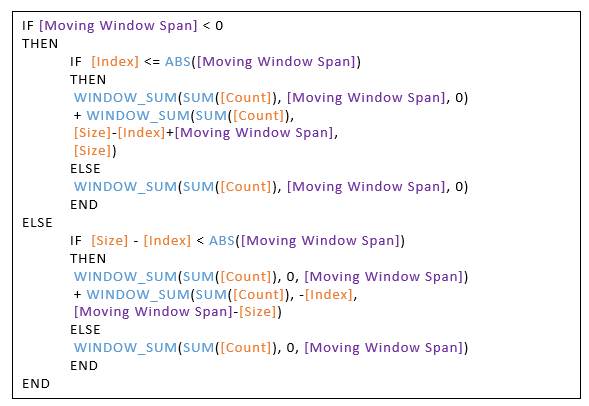Also note that adding of the parameter usage to the default Quick Table calculation, Quick Table Moving Calc (the one without wrapped values) :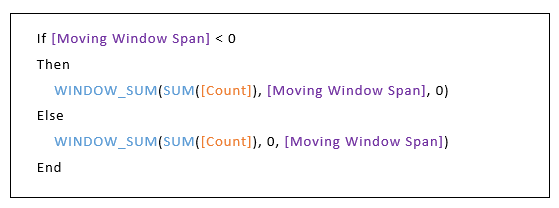You can review this in the workbook linked here.

If the moving calculation requires Previous m and Current value and Next n values, you may need to define an additional parameter and change the calculations accordingly.

It’s time to give it a try yourself!

# Summary

In Moving Calculations with wrap around you will have to include the last few values in the Previous and First in the case of Next to get the right results.

With the help of Index and Size, you will need to manipulate the Table Calculations to consider the otherwise missed values towards first or last few data points.

We hope that this has helped you to set up your first moving calculations with wrap around in Tableau! With this, you will be able to visualize useful insights into subsections of data.

If you need assistance, contact CRGroup, experts in Tableu and business intelligence solutions. crg@crgroup.com